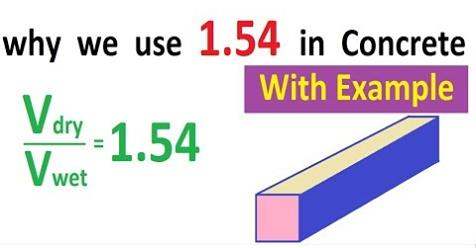What is 1.54 in Concrete || Example solved

# What is 1.54 in Concrete || Example solvedWhat is 1.54 in Concrete
The use of 1.54 in concrete. The ratio of volume of dry concrete to wet concrete is 1.54. Actually the volume of dry concrete is more than the volume of wet concrete. As in the dry condition of concrete, we don't have any water so there are voids present in the concrete and it takes more volume, while in case of the wet concrete we have water present and due to this water there is no voids present in the concrete, so we have a compact mixture of concrete, thus it make volume less of wet concrete, so if know the volume of wet concrete which can be easily calculated in any member, so dry concrete volume can be calculated easily by multiplying the wet concrete with 1.54. Hope it helps in understanding. Volume of dry concrete / Volume of wet concrete = 1.54

Continue Reading about the "What is 1.54 in Concrete || Example solved" on the next page below